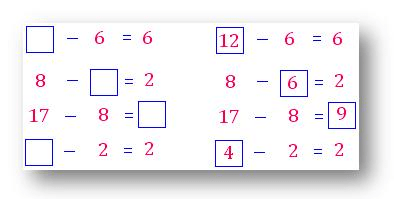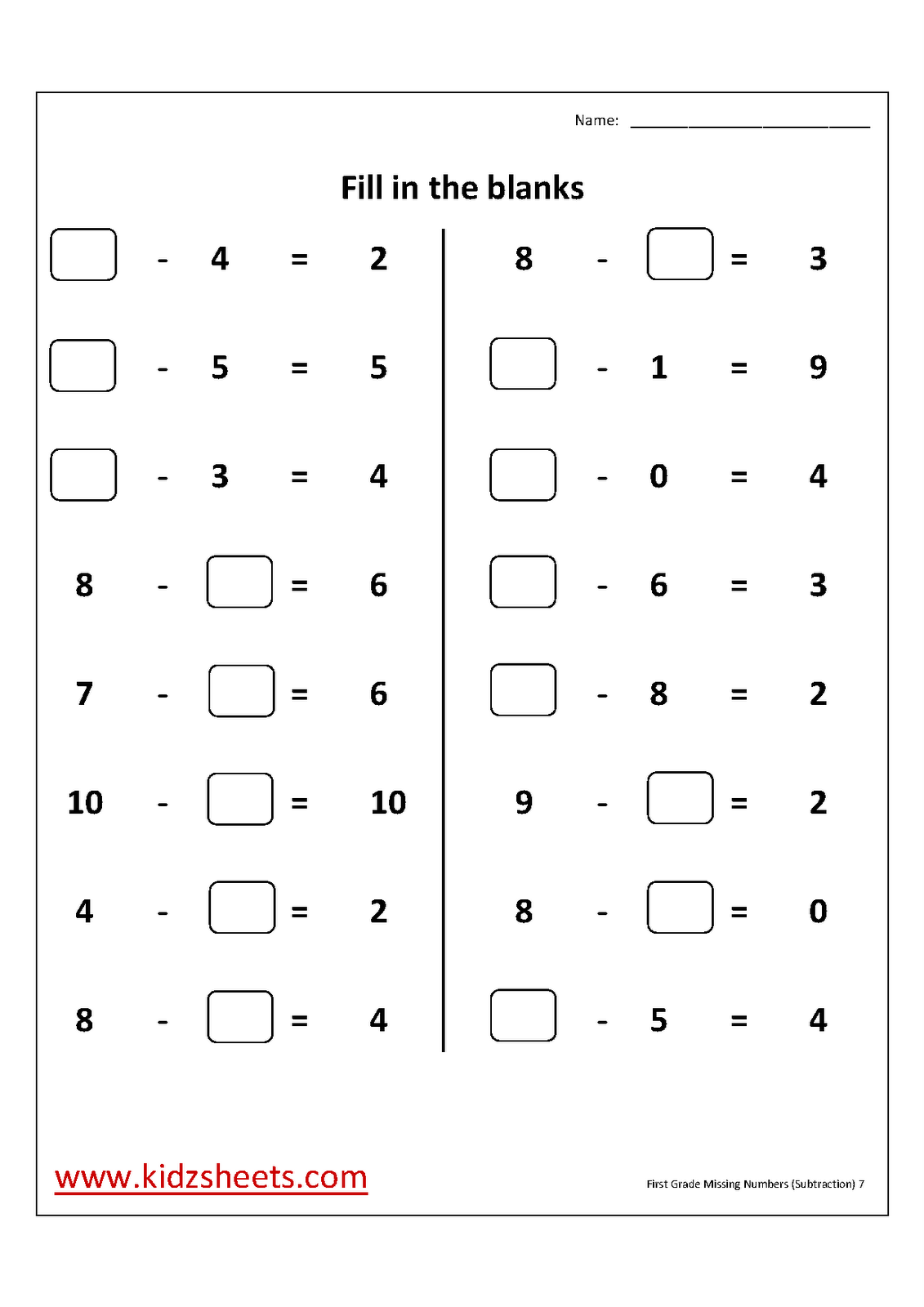# SUBTRACTION

##### Hello children, welcome back to class

SUBTOPIC: OPEN SENTENCES INVOLVING SUBTRACTION

Last week we learnt how to find missing addends in a given maths problem. Now we will learn how to find the missing number in subtraction.

What is the missing number in these subtraction sums?

(i) 9 – ? = 4

What number taken away from 9 leaves 4? The answer is 5.

Therefore, the sum should be written 9 – 5 = 4

(ii) ? – 2 = 5

From what number must you subtract 2 so as to leave 5? The answer is 7. Therefore, the sum should be written 7 – 2 = 5

(iii) 6 – ? = 5

What number taken away from 6 leaves 5? The answer is 1.

Therefore, the sum should be written 6 – 1 = 5.

(iv) ? – 7 = 1

From what number must you subtract 7 so as to leave 1? The answer is 8. Therefore, the sum should be written 8 – 7 = 1

Try the following ActivitiesMORE ACTIVITIESHow Can We Make ClassNotesNG Better? - CLICK to Tell Us Now!